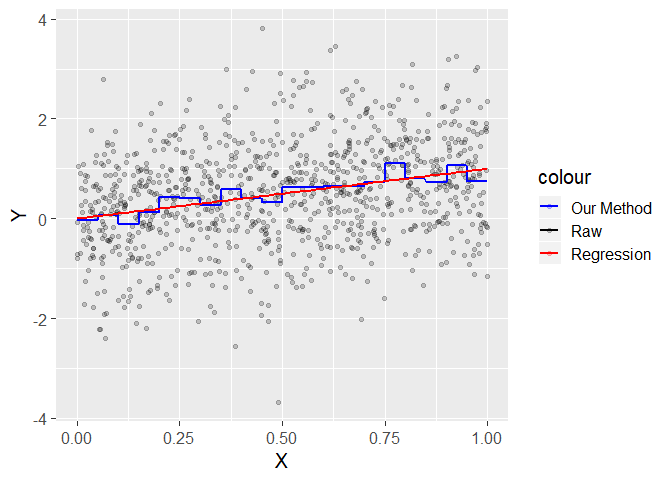# Lecture 28 Explaining Better - Regression Part 2

## Final Exam

• Reminder of good review material: all the homeworks, end-of-lecture practice slides, the midterms
• Today we’ll also be revisiting some of the programming bits we haven’t done in a while
• We did just do a whole review of causal inference, too. May want to check back in on that just in case
• You’ll be fine!

## Today

• We’ll be talking briefly about how all of the causal inference methods we’ve done work in regression
• Plus, we’ll be talking about some ways that people in other fields explain one variable with another
• Just a little peek into what’s out there!
• Then we’ll do a little programming recap

## Causal Inference with Regression

• Remember, regression is about explaining one variable with another by fitting a line
• Typically a straight line, but doesn’t have to be
• So instead of taking a mean within a particular range and having a “stair-step” kind of thing, we have a line that describes what we expect the mean of `Y` to be for any given value of `X`

## Causal Inference with Regression## Causal Inference with Regression

• We’re still explaining, it’s just a different shape. So everything transfers over pretty well!
• You can see how it’s less sensitive to random noise in the data (good, relative to our method), and lets us easily calculate standard errors (good, and something you’ll do in future classes), but is also reliant on the shape that the data takes (bad)
• As another note, when our `X` is a logical or a factor, regression and our method are exactly the same! This will make doing most of our methods easy!

## Fixed Effects

• So, controlling for a factor, or doing fixed effects? No difference (although with regression usually we’d get the effect in the reg, not with correlation)
``````library(Ecdat)
data(Airline)

# Our method
AirlineOurMethod <- Airline %>% group_by(airline) %>%
mutate(output.r = output - mean(output),
cost.r = cost - mean(cost))
AirlineReg <- Airline %>%
mutate(output.reg = residuals(lm(output~factor(airline))),
cost.reg = residuals(lm(cost~factor(airline))))

c(cor(AirlineOurMethod\$output.r,AirlineOurMethod\$cost.r),
cor(AirlineReg\$output.reg,AirlineReg\$cost.reg))``````
``##  0.9272297 0.9272297``

## Difference-in-Differences

• Same with difference-in-difference!
``````load('mariel.RData')
#(some data-cleaning omitted here, see the code for the slides)
#Then we can do our difference in difference with our method
means <- df %>% group_by(after,miami) %>% summarize(lwage = mean(lwage),unemp=mean(unemp))
(means\$lwage - means\$lwage) - (means\$lwage-means\$lwage)

#or by regression, using an "interaction term"
lm(lwage~after*miami,data=df)``````
``##  0.02740653``
``````##
## Call:
## lm(formula = lwage ~ after * miami, data = df)
##
## Coefficients:
##         (Intercept)            afterTRUE            miamiTRUE
##             1.88186             -0.04606             -0.14674
## afterTRUE:miamiTRUE
##             0.02741``````

## Regression Discontinuity

• We saw last time how this works - we fit a line on either side of the cutoff and see how that line jumps at the cutoff